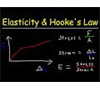Want to see ALL questions on this topic?

Upgrade to PLUS+ for €35 to see all past questionsYou need to have an account to continue

Testing of Materials and Products Videos & Notes

All
Videos
Websites
Notes
1 Videos & Notes
•Elasticity & Hooke's Law - Intro to Young's Modulus, Stress & Strain, Elastic & Proportional Limit
This physics video tutorial provides a basic introduction into elasticity and hooke's law. The basic idea behind hooke's law is that the change in length of a stretching or compressing an object is proportional to the force applied up to the proportional limit. The elastic limit is the point up to which an object can return to its natural state if the external force is removed. Beyond the elastic region lies the plastic region where an object will not return to its natural length if the external force is removed but it experiences permanent deformation. If the force passes the breaking point, the object will snap in two. The ultimate strength is maximum force that an object can withstand without breaking in two. Stress is the ratio of force and area. Stress is the external pressure applied to an object where as strain is the material's response to that stress. Strain is the ratio between the change in length of the object and its original length. The elastic modulus or young's modulus is the ratio between stress and strain. This video provides the equations and formulas needed to perform calculations associated with young's modulus /elastic modulus, stress, and strain. New Physics Video Playlist: https://www.youtube.com/playlist?list=PL0o_zxa4K1BU6wPPLDsoTj1_wEf0LSNeR Access to Premium Videos: https://www.patreon.com/MathScienceTutor https://www.facebook.com/MathScienceTutoring/